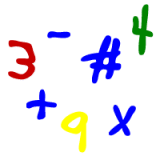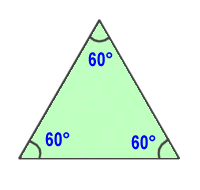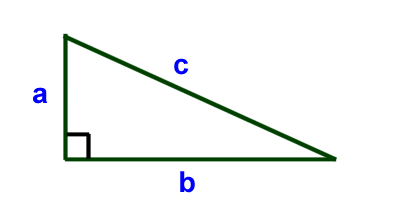Parents and Teachers: Support Ducksters by following us onor.

Kids Math

TrianglesTriangles are three sided polygons. One of the most interesting things about triangles is that if you sum up the three angles within any triangle, they will always total 180 degrees. This comes in handy when working on geometry problems.

There are several types of triangles. Some triangles can be described by their sides, like the equilateral, isosceles, and scalene triangles. Some triangles are described by their angles like the right, obtuse, and acute triangles.

Equilateral Triangle
 The equilateral triangle is a triangle with sides that are all the same length. The three interior angles are all the same too. If we use what we learned above, that all the angles must total 180 degrees, then each angle in an equilateral triangle is 180/3 = 60 degrees.Isosceles Triangle
 An isosceles triangle is a triangle with two equal sides. As a result of having two equal sides, two of the angles will be the same too.Scalene Triangle

A scalene triangle is one where none of the sides are the same length.

Right Triangle

The right triangle is one where one of its angles is 90 degrees. This type of triangle has some unique properties that are important to geometry including the Pythagorean Theorem. Triangles that are not right triangles, or don't have one 90 degree angle, are called oblique.Acute Triangle

An acute triangle is a triangle where all the interior angles are less than 90 degrees.

Obtuse Triangle

An obtuse triangle is the opposite of an acute triangle in that it is a triangle where one angle is greater than 90 degrees.

Acute and obtuse triangles are both oblique.

• Triangles are becoming used more in construction. They are harder to use than rectangles, but can be stronger and may be more resistant to earthquakes.
• If you draw a line in a rectangle from far corner to corner you cut the rectangle into two right triangles.
• If you know two of the angles in a triangle, you can always figure out the third because they must add up to 180 degrees.
• The sum of the lengths of any two sides of a triangle is always longer than the third side.
• The triangle and two is a type of defense used in basketball.
• In North Carolina there are three cities: Raleigh, Durham, and Chapel Hill, that are often referred to as The Triangle.

More Geometry Subjects

Circle
Polygons
Triangles
Pythagorean Theorem
Perimeter
Slope
Surface Area
Volume of a Box or Cube
Volume and Surface Area of a Sphere
Volume and Surface Area of a Cylinder
Volume and Surface Area of a Cone
Angles glossary
Figures and Shapes glossary

Back to Kids Math

Back to Kids StudyFollow us onor